# Sea water

Seawater contains about 4.3% salt. How many dm3 of distilled water we must pour into 5 dm3 of sea water to get water with 1.8% salt?

V =  6.94 dm3

### Step-by-step explanation: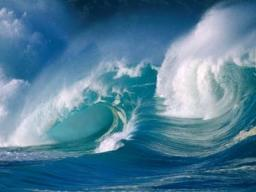Did you find an error or inaccuracy? Feel free to write us. Thank you!Tips to related online calculators
Need help to calculate sum, simplify or multiply fractions? Try our fraction calculator.
Check out our ratio calculator.
Do you have a linear equation or system of equations and looking for its solution? Or do you have a quadratic equation?
Tip: Our volume units converter will help you with the conversion of volume units.
Tip: Our Density units converter will help you with the conversion of density units.

## Related math problems and questions:

• Distilled waterSea water contains 5% salt. How many distilled water should be pour to 40 kg of sea water so that the salt content is 2%. How many kilograms of 2% of sea water we get?
• Sea waterMixing 62 kg of sea water with 84 kg rainwater is created water containing 3.1% salt. How many percent sea water contains salt?
• Vinegar 2How many percentages of vinegar solution will we get if we mix 3.5 liters of 5.8% and 5 liters of 7.6% vinegar?
• AlcoholHow many 55% alcohol we need to pour into 14 liters 75% alcohol to get p3% alcohol? How many 65% alcohol we get?
• Disinfecting solutionHow much distilled water is necessary to pour into 500 ml of 33% hydrogen peroxide solution to obtain 3% disinfecting solution?
• SaltSeawater containing 5% salt. How many kg of freshwater necessary to pour into 40 kg of seawater so that the salinity fell to 2%?
• Vinegar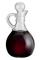We must dilute 16 liters of 8.8% aqueous vinegar to 4.1% one. How much water is necessary to add?
• Peroxide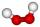How much distilled water (in liters) must pharmacists pour into 300 ml of 23.6% solution of hydrogen peroxide to get 2.7% solution to gargle?
• Vinegar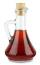What percentage vinegar we get if we mix 1 if dm³ eight percent vinegar with 1.5 dm³ six percent vinegar?
• Sea waterOne tonne of sea water contains 25 kg of salt. How many tonnes of sea water need to be evaporated to obtain 1 tonne of salt?
• Water solutionHow many grams of salt should we dissolve in 400 g of water to get a 20% solution?
• Wood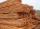Wood contains 12% water. 1 m3 of wood weighs 650 kg. How many liters of water it contains?
• Fertilizer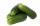Mr. Gherkin use for fertilization 7% solution of fertilizer. 17 liters of it still left. How much water must be added to the solution to make only 3% solution?
• Clogging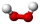How much-distilled water must the pharmacist add to 30g of a 30% hydrogen peroxide solution to obtain a 3% solution to clogging?
• IcerinkRectangular rink with dimensions of 68.7 m and 561 dm must be covered with a layer of ice 4.2 cm thick. How many liters of water is necessary for the formation of ice when the volume of ice is 9.7% greater than the volume of water.
• Salt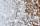1 t of seawater contains 25 kg of salt. How many tons of seawater must be evaporated to obtain 2 q of salt?
• Alcohol mixtureThree liters of 96 percent alcohol set up a certain amount of distilled water to form 54-percent alcohol. How many liters of distilled water was used?